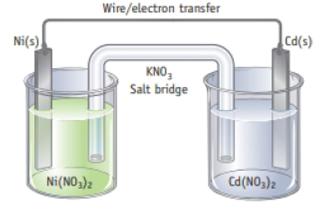# Choose the correct responses based on the cell below in which the following reaction occurs: Ni 2+ (aq) + Cd(s) → Ni(s) + Cd 2+ (aq) 3. Salt bridge (which contains K + and NO 3 − ions): (a) NO 3 − ions move from the Cd half-cell to the Ni half-cell and K + ions move from the Ni half-cell to the Cd half-cell. (b) NO 3 − ions move from the Ni half-cell to the Cd half-cell and K + ions move from the Cd half-cell to the Ni half-cell.### Chemistry & Chemical Reactivity

9th Edition
John C. Kotz + 3 others
Publisher: Cengage Learning
ISBN: 9781133949640

#### Solutions

Chapter
Section### Chemistry & Chemical Reactivity

9th Edition
John C. Kotz + 3 others
Publisher: Cengage Learning
ISBN: 9781133949640
Chapter 19.2, Problem 3RC
Textbook Problem
1 views

## Choose the correct responses based on the cell below in which the following reaction occurs:Ni2+(aq) + Cd(s) → Ni(s) + Cd2+(aq)3. Salt bridge (which contains K+ and NO3− ions): (a) NO3− ions move from the Cd half-cell to the Ni half-cell and K+ ions move from the Ni half-cell to the Cd half-cell. (b) NO3− ions move from the Ni half-cell to the Cd half-cell and K+  ions move from the Cd half-cell to the Ni half-cell.

Interpretation Introduction

Interpretation: The migration of ions in the salt bridge of the given cell is to be determined from the given option.

Concept introduction:

• The substance that easily be reduced in a reaction is represented as an oxidizing agent. For metal cations, a good oxidizing agent can be determined by the standard reduction potential values.
• Half-cell: In the electrochemical cell, both oxidation and reduction occurs. Oxidation occurs at the anode and reduction occurs at the cathode.
• Salt bridge is connected between two half-cells to balance the charge.

### Explanation of Solution

Reason for correct option

Salt bridge is connected between two half-cells to balance the charge. To balance the charge, negative ion in the salt bridge migrates from cathode to anode whereas positive ions migrate from anode to cathode. In the given cell, Ni electrode is cathode and Cd electrode is the anode. Therefore, NO3 ions move from the Ni half-cell to the Cd half-cell and K+ ions moves from the Cd half-cell to the Ni half-cell.

Hence the correct option for the migration of ions in the salt bridge of the given cell is option b.

Reasons for incorrect options:

Write all the given migration of ions in the options except option b

### Still sussing out bartleby?

Check out a sample textbook solution.

See a sample solution

#### The Solution to Your Study Problems

Bartleby provides explanations to thousands of textbook problems written by our experts, many with advanced degrees!

Get Started

Find more solutions based on key concepts
0 Explain the relationship between transition-state analogs and abzymes.

Introduction to General, Organic and Biochemistry

Why is the air clear above the inversion layer?

Fundamentals of Physical Geography

Moderate alcohol use has been credited with providing possible health benefits. Construct an argument for why m...

Nutrition: Concepts and Controversies - Standalone book (MindTap Course List)

Where are protons, electrons, and neutrons found in an atom?

Biology: The Dynamic Science (MindTap Course List)

A dive-bomber has a velocity or 280 m/s at ail angle below the horizontal. When the altitude of the aircraft i...

Physics for Scientists and Engineers, Technology Update (No access codes included)

Describe the law of hydrostatic equilibrium.

Foundations of Astronomy (MindTap Course List)